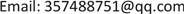1. 引言

Training data se

r1 华北路东、西南路北AB区、东规划路、西华北路、南西南路、北规划路121.57、38.94沙河口区春风街1号、西南路905-1号~905-7号、春风街5-1号
r2 沙河口区春风街1号、西南路北侧A、B区、西南路905-1号华北路东、东至规划路、 春风街5-1号、西南路905-7号121.575、39.945、南西南路、 西华北路、北规划路

1) 提出一种不考虑模式、类型的记录相似度定义，能够有效的表达半结构化记录的相似性。

2) 在本文定义的字符串相似度基础上，引入最大二分图加权匹配算法(Kuhn-Munkres算法)有效计算记录之间的相关性。

3) 为了减少候选记录之间的比较次数，引入并改进集合相似性，为记录创建有效签名空间，以及生成最优签名的步骤，将签名和倒排索引结合，对相关记录进行初步选择。

4) 为了降低最大匹配算法的时间复杂度，采用两种新的过滤器，减少不相关的候选集，同时采用三角不等式来优化最大匹配方法。

2. 相关工作

3. 相关理论3.1. 字符串相似度

N E d s ( x , y ) = 1 − E D ( x , y ) max ( | x | , | y | )

C ( S ) = ∑ i = 1 k C (ei)

E D ( x , y ) = min { C ( S ) }

3.2. 集合相关性

Φ α ( x , y ) = { Φ ( x , y )       if   Φ ( x , y ) ≥ α 0                           if   Φ ( x , y ) ≤ α

1) SET-SIMILARITY：检查两个集合是否相似。则SET-SIMILARITY计算公式为：

r e l a t e d Φ ( R , S ) = s i m i l a r Φ α ( R , S ) = | R ∩ ˜ Φ α S | | R | + | S | − | R ∩ ˜ Φ α S |

2) SET-CONTAINMENT：检查一个集合是否是另一个集合的子集。其中 | R | ≤ | S | ，则SET-CONTAINMENT的计算公式为：

r e l a t e d Φ ( R , S ) = c o n t a i n Φ α ( R , S ) = | R ∩ ˜ Φ α S | | R |

4. 基于字符串相似度的实体解析算法4.1. 数据分词

Training data set string representatio
r1沙河口区春风街1号、西南路905-1号~905-7号、春风街5-1号、华至北路东、 西南路北A B区、东至规划路、西至华北路、南至西南路、北至规划路、121、39
r2春风街1号、西南路北侧、西南路905-1号、华北路东、东至规划路、 春风街5-1号、西南路905-7号、121.575、39.945、南西南路、西华北路、北规划路

Data segmentation result
r1r2

121、39西华北路

4.2. 记录相关性计算

E ′ ⊂ E 。则记录ri和rj的相似度定义为： S i m ( r i , r j ) = ∑ e ∈ E ′ W ( e ) max { m , n } 其中m、n分别代表ri和rj的子字符串个数。

1) 由算法1构造两条记录的完全二分图；

2) 算法2计算两条记录相似性，其中 s i m i l a r i t y ( r 1 [ i ] , r 2 [ j ] ) 返回r1的第i个字符串和r2的第j个字符串之间的相似性， o p t i m a l _ m a t c h ( B , w , m ) 指的是二分图的最优匹配，返回最优匹配中所有权值之和，其中B表示完全二分图，w是B的权值矩阵，m是w的阶；

3) 根据用户需求给定阈值 δ ，用算法3将记录之间相似度大于该阈值的记录选择出来作为相似记录对。

5. 基于集合相关性的实体解析算法

5.1. 属性值规范化标记分词及创建倒排索引表

Semi-structured tag array collection representatio
R
r1: (t1 = 西南路， t5 = A、B区， t6 =沙河口区， t4 =春风街， t7 = 1号， t8 = 905-1号) r2: (t1 = 西南路， t2 = 华北路， t3 = 规划路， t4 = 春风街， t9 = 5-1号， t10 = 905-7号) r3: (t1 = 西南路， t2 = 华北路， t3 = 规划路， t11 = 121.57， t12 = 38.94)

5.2. 有效签名生成

5.2.1. 有效签名

5.2.2. 加权签名方案

c o n t a i n ∅ ( R , S ) = | R ∩ ˜ ∅ S | | R | ≥ δ

s i m i l a r ∅ ( R , S ) = | R ∩ ˜ ∅ S | | R | + | S | − | R ∩ ˜ ∅ S | ≥ δ

δ ≤ | R ∩ ˜ ∅ S | | R | + | S | − | R ∩ ˜ ∅ S | ≤ | R ∩ ˜ ∅ S | | R | + | S | − | S | = | R ∩ ˜ ∅ S | | R |

∑ i = 1 n | r i | − | k i | | r i | = 6 − 1 6 + 6 − 2 6 + 5 − 2 5 = 2.1 < θ 。

5.2.3. 最优签名选择

∑ t ∈ K R T | I [ t ] | 最小化， | I [ t ] | 指的是倒排索引表中标记的个数。

1) 给出一个集合 R = { r 1 , r 2 , ⋯ , r n } ，对于每一个在 R T 中的t，给它赋值 value = ∑ r i | t ∈ r i 1 | r i | 以及一个代价 cost = | I [ t ] | ；

2) 为了最小化倒排序列的大小，在 R T 中按cost/value对所有标记以递增次序进行排序；

3) 依次选择标记，直到定义7中的条件得到满足为止，得到有效签名，其中所选的标记是 K R T 的签名标记；

5.3. 候选集过滤

5.3.1. 检查过滤器

5.3.2. 最近邻过滤器

| R ∩ ˜ ∅ S | ≤ ∑ r ∈ R max s ∈ S Φ ( r , s )

1) 搜索有效最近邻：遍历每个标记 t ∈ r ，对于每一个标记t，使用倒排索引表I[t]获取S中包含标记t的所有元素s。计算得到r和每个s之间的相似性；相似度得分最大的s被认为是最近邻。

2) 计算重用：在检查过滤器中，已经计算了r和 s ∈ S 中包含r的签名标记的所有元素之间的实际相

3) 提前终止：对于 r ∈ R 中签名标记出现在候选集合S中的元素，其最近邻相似度并不能保证

Φ ( r , s ) ≤ | r | − | k | | r | ，因此要么重用检查过滤器中的计算公式，要么直接进行最近邻搜索。而对于签名标记没有出现在S集合中的元素 r ′ ∈ R ，对所有 s ∈ S 边界 | r ′ | − | k ′ | | r ′ | 仍然保留。该算法首先通过对匹配元素r的最近邻相似性进行求和来推断出一个总的估计值，并使用 | r ′ | − | k ′ | | r ′ | 估计非匹配元素 r ′ 。然后，遍历每个 r ′ ，使用 r ′ 的最近邻相似性来更新总估计值。如果总估计低于θ，则删除该候选集。

5.4. 最大匹配验证

ϕ ( r , s ′ ) + ϕ ( r ′ , s ) = 1 − ψ ( r , s ′ ) + 1 − ψ ( r ′ , s ) (1)

= 2 − ψ ( r , s ′ ) − ψ ( r ′ , r ) (2)

≤ 2 − ψ ( r ′ , s ′ ) (3)

= 1 + 1 − ψ ( r ′ , s ′ ) (4)

= ϕ ( r , s ) + ϕ ( r ′ , s ′ ) (5)

6. 实验评估6.1. 数据集

Statistics of information extracted from real estat

6.2. 实验方法

Description of experimental method
NameDescription

6.3. 实验结果及分析

7. 总结